#The Model Student: A Primal Skyline (Part 2)

Overload Journal #93 - October 2009 + Programming Topics   Author: Richard Harris
How do you measure the length of a curve? Richard Harris gets his ruler out.

In the previous article I described some of the important questions regarding the lone wolves of the number line, the prime numbers; those integers greater than 1 not wholly divisible by any positive integer other than themselves and 1.

We discussed Euclid's elegant proof that there are infinitely many of them and described the prime number theorem which gives an approximate estimate of π; the function that counts the number of primes less than or equal to any given integer.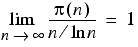Recall that the lim term denotes the limit that the following term converges to 1 as n grows ever larger.

Given that greater minds than mine have tried and failed to find the precise pattern by which the prime numbers are found within the integers, I suggested that we look at the prime factorisations of the integers instead. Every positive integer can be uniquely represented by a prime factorisation, the collection of prime numbers that when multiplied together yield the integer in question.

The number 42, for example, has the prime factors 2, 3, and 7 since 42 = 2 × 3 × 7.

The number 1 is, as you will no doubt recall, the special case of multiplying no primes together.

Rather than completely analyse the prime factorisations of every integer, we instead took a look at one of π's relatives, the function that counts the number of prime factors (including repeated factors) of a given integer, Ω, shown in Figure 1.

nΩ(n)
0-∞
10
21
31
42
51
62
nΩ(n)
71
83
92
102
111
123
131
nΩ(n)
142
152
164
171
183
191
203
Figure 1

Noting that 0 can be considered the result of dividing 1 by an infinite number of prime factors, figure 1 gives the values of Ω for the integers between 0 and 20.

Figure 2 reproduces the graphs of Ω for the integers from 1 to 20 (top) and 1 to 100 (bottom).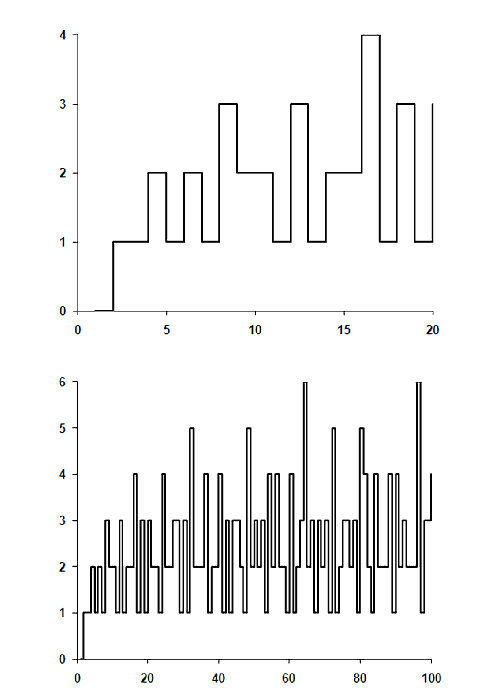Figure 2

In pursuit of a pattern in these graphs, we introduced our own function ףn, defined by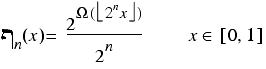Recall that the odd brackets surrounding the 2nx term mean the largest integer less than or equal to the value between them and that the expression on the right with the rounded E mean that x lies between 0 and 1.

Two example graphs of ףn‎5 on the left and ף‎7 on the right) are given in figure 3.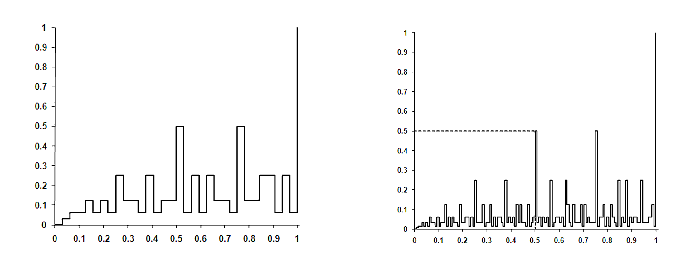Figure 3

Finally, we demonstrated that successive versions of ףn are coincident for half the points in the range 0 to 1 and that the infinite limit, ף‎∞, sort of exhibits the property of self similarity since it can be entirely recovered from an arbitrarily small range of arguments.

Both of these properties bear a striking resemblance to those of fractals and we closed on the question of whether ף‎∞ is itself a fractal. Before attempting to answer that question, I think it would be prudent to define precisely what a fractal is.

### OK, so what precisely is a fractal?

Beloved of undergraduate mathematicians the world over, fractals hit mainstream popular culture in the late 1980s. In fact, I still have a T-shirt with a black and white print of a fractal. Made from hemp. As is tragically illustrated in figure 4: Dear God, what was I thinking?[Asti].Figure 4

Whilst these beautiful images may have adorned the torsos of many of my fellow unwashed hippies, I rather suspect that their exact definitions were not so widely disseminated.

Strictly speaking, a fractal is a curve, area, volume or higher dimensional analogue that has a non-integer, or in other words fractional, dimension. A somewhat counter-intuitive description, it relies upon a very particular definition of dimension; that of fractal dimension. There are many different types of fractal dimension and we shall focus on one of the simplest to calculate, the Minkowski, or box-counting dimension.

For a curve, this is a measure of how quickly its length grows as the ruler you use to measure it shrinks.

Specifically, if a ruler of length e can be lain end to end N(e) times to cover a curve, then its box-counting dimension is defined as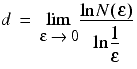Note that the term to the left of the fraction means that we should consider the limit of the term to its right as ε tends to 0.

### The Koch curve

To demonstrate the idea, we introduce the Koch curve. This curve is iteratively constructed from the element illustrated in figure 5.Figure 5

The first iteration in the construction of the Koch curve is to replace each of the 4 straight line segments with copies of the basic element shrunk in length by 1/3, as illustrated in figure 6.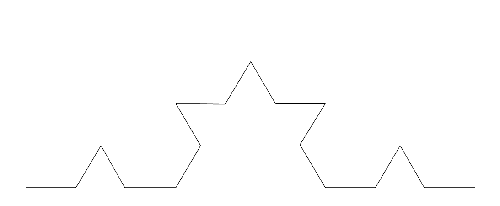Figure 6

We continue in the same vein for further iterations, ever replacing the straight line segments with further and further scaled down copies of the basic element.

Figure 7 illustrates the result of a few more iterations in the construction of the curve.Figure 7

Assuming that the curve ranges from 0 to 1 on the x axis, a ruler of length 1/3 can be laid end to end on the curve 4 times; at this scale it appears identical to the basic element. Similarly, to a ruler of length 1/9, the curve appears identical to the first iteration and can be covered with 16 of them.

Generalising this, to a ruler of length 1/3n the curve appears identical to the n-1th iteration of its construction and, since at each iteration we replace each line segment with 4 lines each 1/3 its length, will therefore be covered by 4n of them.

The dimension of the Koch curve under this definition is therefore approximately 1.2619 as shown in derivation 1.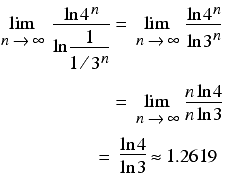Note that we take the limit as n tends to infinity, which since our ruler is of length 1/3n is equivalent to the ruler length tending to 0 and the length of the curve tending to infinity. Derivation 1

## The fractal dimension of a straight line

One thing we should check is that this measure of dimension yields the correct value of 1 for simple curves such as, for example, the straight line from (0,0) to (1,1). The length of this line is √2 and so, for a ruler of length ε, we will need √2/ε of them to cover the line.

The dimension of this line using this measure is indeed 1, as demonstrated in derivation 2.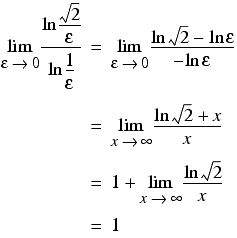Derivation 2

## The fractal dimension of the circumference of a circle

For a more complex example, consider the circumference of a circle with radius 1 centred at the origin. If we choose our ruler lengths carefully, we can join points on the circle to construct inscribed regular polygons of increasing numbers of sides, as illustrated in figure 8.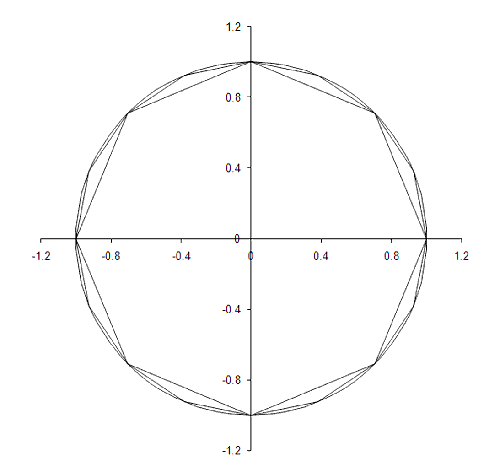Figure 8

We can see from this diagram that as we increase the number of sides of the inscribed polygon we both move closer and closer to the circle and make smaller and smaller corrections to the previous polygonal approximation. This is highly suggestive that the fractal dimension is 1, although proving it is a little more tricky, as illustrated in derivation 3.

 The circumference of a polygon with n sides is trivially n times the length of each side, which for our measure of the circumference is equal to the length of the ruler, εn, and which we can recover with a little trigonometry.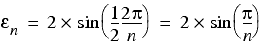As n tends to infinity, so the length of our ruler, εn, tends to 0, enabling us to express the box-counting dimension asAs n grows ever larger so π/n grows ever smaller and we can approximate sin(π/n) with ever increasing accuracy using a few terms of a Taylor's series expansion in which a function is represented by a polynomial with coefficients calculated from its derivativesHere we use 1 dash to mean the first derivative, 2 dashes to mean the second and (n) to mean the n'th, and the exclamation mark stands for the factorial of the number preceding it, which is equal to the product of every number from 1 up to and including that number. Using just the first 2 terms with a equal to 0 to approximate the sine function we have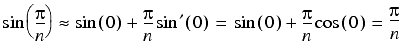The limit of our box-counting measure of the dimension of the circumference of the unit circle now becomes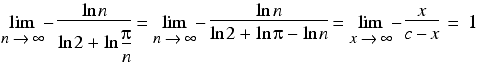Derivation 3

Note that for areas, volumes and higher dimensional objects a similar approach is used in which we cover the object of interest in 2 dimensional patches of ever decreasing area, 3 dimensional blocks of ever decreasing volume and so on.

## The fractal dimension of ף‎∞

One of the principal advantages of the box-counting measure of fractal dimension is that it is supremely simple to implement as a numerical algorithm. And since I wouldn't even know how to start calculating the fractal dimension of ף‎∞ mathematically, this is going to prove rather handy.

We have a natural choice for the ruler length for ףn, a new definition of εn given by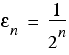since each horizontal line in the graph is exactly this long, and each vertical line has a length equal to an integer multiple of this, namely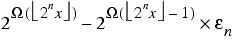The straight line braces in this formula denote the absolute value of the expression between them; the positive number with the same magnitude, or size, as the value of the expression, or equivalently, the strictly positive distance between it and 0.

Note that the admissible values of x will be precisely those for which the graph has a vertical line; those that are equal to an integer multiplied by εn.

## An upper bound for N(εn)

Before we start, however, we shall need an upper bound on the number of rulers of length εn required to cover the graph of ףn, since we need to ensure that the type we use to count them is large enough.

A simple curve that is guaranteed to be no shorter than ףn is one in which for every number greater than or equal to 2iεn and less than 2i+1εn is associated with a vertical line of length 2iεn. The reason that this is an upper bound is that above 0, there is no number that has negative infinity factors or as many factors as the smallest power of 2 greater than or equal to it. Hence at every point at which both curves have a vertical line that of the upper bound curve must be the longer.

We construct this curve by taking a value of 1 at 0 and then dropping to 0 and rising to the relevant power of 2 of εn at alternate steps of εn.

Figure 9 illustrates this curve for ף‎4 in units of ε4.Figure 9

The number of εn length rulers required to cover the horizontal lines in this graph is trivially 2n. For the vertical lines, the calculation is a little more complicated and results in approximately 22n-1 as shown in derivation 4.

 Considering the ף‎4 case will give us a hint as to how we might go about counting the number of rulers. The length of the vertical lines in this curve in units of ף‎4 is given by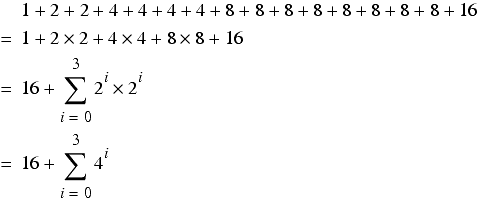In general, the length of the vertical lines in the upper bound curve for ףn is given byand hence the total length of the upper bound curve issince the sum is another example of a geometric series. Derivation 4

Despite this being a gross overestimate of the actual number of rulers we'll need to cover the curve, we can be fairly sure that if we use inbuilt types we shall overflow them in short order.

It seems reasonable, therefore, to create an accumulator type of our own that won't suffer from this problem, one that I shall imaginatively call accumulator and whose definition is provided in listing 1.

 ``` class accumulator { public: accumulator(); accumulator & operator+=(unsigned long n); operator double() const; private: std::vector sum_; }; ``` Listing 1

The constructor is pretty trivial; we simply initialise the sum_ with a single 0 as shown below:

```      accumulator::accumulator() : sum_(1, 0) {}
```

The operator+= member function requires a little more thought however.

Recall that the C++ standard declares that arithmetic with unsigned integer types cannot overflow and that arithmetic using n bit unsigned integer types is performed modulo 2n [ANSI]. Any bits in the result of an arithmetic expression that don't fit into the unsigned integer type are simply discarded and the result wraps around into the type's valid range.

This is actually quite useful for us, since we'll be keeping track of the discarded bits ourselves and the correct value for our lowest unsigned long digit is exactly the wrapped around result of the addition.

Noting that when an addition wraps around the result must be less than the value that we are adding to our accumulator, listing 2 illustrates the implementation of the in place addition operator.

 ``` accumulator & accumulator::operator+=(unsigned long n) { assert(!sum_.empty()); sum_.front() += n; if(sum_.front()::iterator first = sum_.begin(); std::vector::iterator last = sum_.end(); while(++first!=last && ++*first==0); if(first==last) sum_.push_back(1); } return *this; } ``` Listing 2

Since an addition can only ever add 1 to the next most significant digit, the loop we enter upon wrapping around simply checks whether incrementing subsequent digits by 1 also wrap around, pushing a 1 onto the end in the event that they all do.

The conversion to double is much simpler, as shown in listing 3. Here we simply add up the elements of our accumulator as doubles of increasing magnitude. It's not as efficient as it might be since later digits may cause the earlier ones to round off due to the limited precision of the double type, meaning that some of the earlier calculations may be wasted.

 ``` accumulator::operator double() const { static const int dig = std::numeric_limits::digits; static const double base = pow( 2.0, double(dig)); std::vector:: const_iterator first = sum_.begin(); std::vector:: const_iterator last = sum_.end(); double result = 0.0; double mult = 1.0; while(first!=last) { result += mult * double(*first++); mult *= base; } return result; } ``` Listing 3

We shall rarely use the conversion though, so it's really not worth making the code more complicated to improve its efficiency.

So now we have all the pieces in place to calculate the box-counting dimension of ףn. We will reuse the count_factors from the last article as illustrated again in listing 4.

 ``` template unsigned long count_factors(unsigned long x, FwdIt first_prime, FwdIt last_prime) { unsigned long count = 0; while(first_prime!=last_prime && (*first_prime)*(*first_prime)<=x) { while(x%*first_prime==0) { ++count; x /= *first_prime; } ++first_prime; } if(x>1) ++count; return count; } ``` Listing 4

Recall that if a number has precisely 1 factor it is, by definition, prime and we shall use this fact to update the sequence of primes whose first and last iterators we'll pass to subsequent calls to the count_factors function.

This time, however, we shall wish to push on to the very limits of unsigned long and so shall need to take care that we do not fall foul of the wrap around problems we identified in the previous part of this article.

We shall do this by adding primes to our list when they are less than or equal to the square root of the upper bound, or as it turns out the square root of 1 less than it, rather than when their squares are less than or equal to the upper bound itself.

## Newton's method for the integer square root

We shall calculate the integer square root using Newton's method for finding arguments at which functions return a value of 0, technically known as roots.

Newton's method is an iterative algorithm in which an initial estimate for a root is repeatedly updated using the formula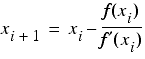where the dash after the f in the denominator indicates the derivative with respect to its argument.

At each step, Newton's method finds the root of a linear approximation of the function in question, as demonstrated in derivation 5.

 Using the first two terms of Taylor's expansion as an approximation of f we have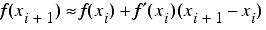Solving for f(xi+1) equal to 0 yields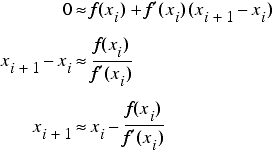Derivation 5

Newton's method can fail to converge under certain conditions, but thankfully these only arise at 0 when using it to calculate square roots.

The equation whose roots are the square roots of a given number a is simply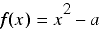The first derivative of this function is 2x, meaning that Newton's method for finding the square root uses the iterative formulaI'm sure that some of you will have come across this algorithm for computing square roots before, although you may not have seen its derivation. I first learnt it from a book of mathematical tricks for the primitive calculators of my youth, although I'm afraid I cannot remember its name.

We implement this with the isqrt function, given in listing 5.

 ``` unsigned long isqrt(unsigned long n) { if(n==0) return 0; unsigned long a0 = 2; unsigned long a1 = n/2; do { a0 = (a0+a1)/2; a1 = n/a0; } while((a01) : (a0-a1>1)); return (a0+a1)/2; } ``` Listing 5

Note that we treat 0 as a special case and that rather than maintain the estimate of the square root, we maintain the two terms that are averaged to retrieve it, and that the iteration stops when these two terms differ by no more than 1. At this point, therefore, one of them must be no larger than the square root plus 1 and the other no smaller than the square root minus 1. Taking one final step ensures that we round down and can thus guarantee that we choose the largest integer less than or equal to the root.

We can be sure that we won't round down from the correct value for the square root when it happens to be an integer, since the second term is simply the argument divided by the first and so if one term is equal to the root, the other must be also.

That I have bothered to implement an integer square root function rather than casting to floating point and using the inbuilt square root function might come as something of a surprise. In my defence I can assure you that, at least on my machine, it is significantly faster. I cannot unfortunately adequately explain why; I rather suspect it has something to do with stalling the processor pipeline.

## Counting the number of rulers

This time rather than print out the values of ףn as we iterate over the integers, we shall accumulate the number of rulers of length en required to cover their graphs and, if we have truly eliminated any possible overflow, we shall be able to do so for every n up to the number of bits in an unsigned long.

Recasting our formula for ףn in terms of our ruler's length, εn, we have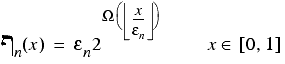since εn is just 1 divided by 2n. The vertical lines in its graph must lie at points where x is an integer multiple of εn and will have length equal to the positive difference in the function's value at that point and its value at the previous integer multiple of εn.

Noting that at 0, ףn has a value of 0, and that every subsequent vertical line is preceded by a horizontal line of length εn, the total length of the graph must be equal to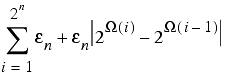The number of rulers of length εn required to cover the graph is therefore this expression divided by εn:A C++ implementation of this calculation, named count_rulers, is presented in listing 6.

 ``` double count_rulers(unsigned long n) { static const int dig = std::numeric_limits::digits; if(n>dig) throw std::invalid_argument(""); const unsigned long upper_bound = ((n==dig) ? 0UL : (1UL< primes; unsigned long prev = 0; unsigned long i = 0; while(i!=upper_bound) { ++i; const unsigned long f = count_factors(i, primes.begin(), primes.end()); const unsigned long curr = 1UL<prev) ? curr-prev : prev-curr; if(i<=max_prime && f==1) primes.push_back(i); acc += 1; acc += len; prev = curr; } acc += 1; acc += upper_bound+1-prev; return double(acc); } ``` Listing 6

Once again, we've had to jump through several hoops to ensure that we can never encounter integer wrap around, as enumerated below.

Firstly, we bail out with an exception if n exceeds the number of bits in an unsigned long, since this is the maximum value for which we will be able to represent every non-negative integer less than 2n.

Secondly, we set the upper bound of the iteration to 2n-1, rather than 2n, since the latter might be equal to 0. Note that we must treat n equal to the number of bits in an unsigned long as a special case since the shift operator irritatingly results in undefined behaviour if we shift by that many bits. I had failed to account for this in my original treatment and my compiler exacerbated my error by both failing to warn me and by doing exactly what I expected. Fortunately the ACCU conference delegates were far more standards compliant and pointed out my mistake. It just goes to show how fiddly dealing with numeric types can be.

Thirdly, we use an integer square root rather than the square of the current integer to determine whether an integer with just 1 factor is small enough to be added to our list of primes. Once again 2n might be equal to 0 so we're forced to use the square root of 2n-1 instead. For n greater than 2, this always yields the correct upper bound since in these cases the square root of 2n is a compound number; specifically a power of 2. Fortunately, for n equal to 2 or less, every number less than 2n must be 0, 1 or prime, so we won't actually need the list of primes during the calculation.

Finally, since we have not included the final term of the sum during the iteration, we add it after the iteration has completed. For any n greater than 0, we can be sure that the penultimate term of ףn is not equal to 0, so the final addition to our accumulator must involve a number strictly less than 2n.

Phew!

So are we finally ready to calculate the fractal dimension of ף‎∞?

Well yes, dear reader, at long last we are. However, I have unfortunately run out of space and so the final analysis shall have to wait until next time.

## Acknowledgements

With thanks to Stewart Brodie for pointing out my undefined use of the shift operator and to John Paul Barjaktarevic and Lee Jackson for proof reading this article.

## References and further reading

[ANSI] The C++ Standard, American National Standards Institute, 1998.

[Asti] Thanks Asti. I think.